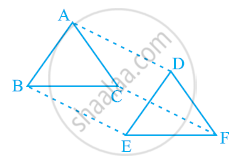# In ΔABC and ΔDEF, AB = DE, AB || DE, BC = EF and BC || EF. Vertices A, B and C are joined to vertices D, E and F respectively (see the given figure). Show that - Mathematics

In ΔABC and ΔDEF, AB = DE, AB || DE, BC = EF and BC || EF. Vertices A, B and C are joined to vertices D, E and F respectively (see the given figure). Show that

(i) Quadrilateral ABED is a parallelogram

(ii) Quadrilateral BEFC is a parallelogram

(iv) Quadrilateral ACFD is a parallelogram

(v) AC = DF

(vi) ΔABC ≅ ΔDEF.#### Solution

(i) It is given that AB = DE and AB || DE.

If two opposite sides of a quadrilateral are equal and parallel to each other, then it will be a parallelogram.

Therefore, quadrilateral ABED is a parallelogram.

(ii) Again, BC = EF and BC || EF

Therefore, quadrilateral BCEF is a parallelogram.

(iii) As we had observed that ABED and BEFC are parallelograms, therefore

(Opposite sides of a parallelogram are equal and parallel)

And, BE = CF and BE || CF

(Opposite sides of a parallelogram are equal and parallel)

(iv) As we had observed that one pair of opposite sides (AD and CF) of quadrilateral ACFD are equal and parallel to each other, therefore, it is a parallelogram.

(v) As ACFD is a parallelogram, therefore, the pair of opposite sides will be equal and parallel to each other.

∴ AC || DF and AC = DF

(vi) ΔABC and ΔDEF,

AB = DE (Given)

BC = EF (Given)

AC = DF (ACFD is a parallelogram)

∴ ΔABC ≅ ΔDEF (By SSS congruence rule)

Concept: Another Condition for a Quadrilateral to Be a Parallelogram
Is there an error in this question or solution?
Chapter 8: Quadrilaterals - Exercise 8.1 [Page 147]

#### APPEARS IN

NCERT Class 9 Maths